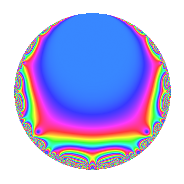Properties

 Label 27.3.dLevel 27 Weight 3 Character orbit d Rep. character $$\chi_{27}(8,\cdot)$$ Character field $$\Q(\zeta_{6})$$ Dimension 2 Newforms 1 Sturm bound 9 Trace bound 0

Related objects

Defining parameters

 Level: $$N$$ = $$27 = 3^{3}$$ Weight: $$k$$ = $$3$$ Character orbit: $$[\chi]$$ = 27.d (of order $$6$$ and degree $$2$$) Character conductor: $$\operatorname{cond}(\chi)$$ = $$9$$ Character field: $$\Q(\zeta_{6})$$ Newforms: $$1$$ Sturm bound: $$9$$ Trace bound: $$0$$

Dimensions

The following table gives the dimensions of various subspaces of $$M_{3}(27, [\chi])$$.

Total New Old
Modular forms 18 6 12
Cusp forms 6 2 4
Eisenstein series 12 4 8

Trace form

 $$2q$$ $$\mathstrut +\mathstrut 3q^{2}$$ $$\mathstrut -\mathstrut q^{4}$$ $$\mathstrut -\mathstrut 6q^{5}$$ $$\mathstrut -\mathstrut 2q^{7}$$ $$\mathstrut +\mathstrut O(q^{10})$$ $$2q$$ $$\mathstrut +\mathstrut 3q^{2}$$ $$\mathstrut -\mathstrut q^{4}$$ $$\mathstrut -\mathstrut 6q^{5}$$ $$\mathstrut -\mathstrut 2q^{7}$$ $$\mathstrut -\mathstrut 12q^{10}$$ $$\mathstrut +\mathstrut 3q^{11}$$ $$\mathstrut +\mathstrut 4q^{13}$$ $$\mathstrut -\mathstrut 6q^{14}$$ $$\mathstrut +\mathstrut 11q^{16}$$ $$\mathstrut +\mathstrut 22q^{19}$$ $$\mathstrut +\mathstrut 6q^{20}$$ $$\mathstrut +\mathstrut 3q^{22}$$ $$\mathstrut +\mathstrut 48q^{23}$$ $$\mathstrut -\mathstrut 13q^{25}$$ $$\mathstrut +\mathstrut 4q^{28}$$ $$\mathstrut -\mathstrut 78q^{29}$$ $$\mathstrut -\mathstrut 32q^{31}$$ $$\mathstrut -\mathstrut 27q^{32}$$ $$\mathstrut -\mathstrut 27q^{34}$$ $$\mathstrut -\mathstrut 68q^{37}$$ $$\mathstrut +\mathstrut 33q^{38}$$ $$\mathstrut +\mathstrut 30q^{40}$$ $$\mathstrut +\mathstrut 21q^{41}$$ $$\mathstrut +\mathstrut 61q^{43}$$ $$\mathstrut +\mathstrut 96q^{46}$$ $$\mathstrut +\mathstrut 84q^{47}$$ $$\mathstrut +\mathstrut 45q^{49}$$ $$\mathstrut -\mathstrut 39q^{50}$$ $$\mathstrut +\mathstrut 4q^{52}$$ $$\mathstrut -\mathstrut 12q^{55}$$ $$\mathstrut +\mathstrut 30q^{56}$$ $$\mathstrut -\mathstrut 78q^{58}$$ $$\mathstrut -\mathstrut 87q^{59}$$ $$\mathstrut -\mathstrut 56q^{61}$$ $$\mathstrut -\mathstrut 142q^{64}$$ $$\mathstrut -\mathstrut 24q^{65}$$ $$\mathstrut +\mathstrut 31q^{67}$$ $$\mathstrut +\mathstrut 27q^{68}$$ $$\mathstrut +\mathstrut 12q^{70}$$ $$\mathstrut +\mathstrut 130q^{73}$$ $$\mathstrut -\mathstrut 102q^{74}$$ $$\mathstrut -\mathstrut 11q^{76}$$ $$\mathstrut -\mathstrut 6q^{77}$$ $$\mathstrut -\mathstrut 38q^{79}$$ $$\mathstrut +\mathstrut 42q^{82}$$ $$\mathstrut +\mathstrut 84q^{83}$$ $$\mathstrut -\mathstrut 54q^{85}$$ $$\mathstrut +\mathstrut 183q^{86}$$ $$\mathstrut +\mathstrut 15q^{88}$$ $$\mathstrut -\mathstrut 16q^{91}$$ $$\mathstrut -\mathstrut 48q^{92}$$ $$\mathstrut +\mathstrut 84q^{94}$$ $$\mathstrut -\mathstrut 66q^{95}$$ $$\mathstrut +\mathstrut 115q^{97}$$ $$\mathstrut +\mathstrut O(q^{100})$$

Decomposition of $$S_{3}^{\mathrm{new}}(27, [\chi])$$ into irreducible Hecke orbits

Label Dim. $$A$$ Field CM Traces $q$-expansion
$$a_2$$ $$a_3$$ $$a_5$$ $$a_7$$
27.3.d.a $$2$$ $$0.736$$ $$\Q(\sqrt{-3})$$ None $$3$$ $$0$$ $$-6$$ $$-2$$ $$q+(1+\zeta_{6})q^{2}-\zeta_{6}q^{4}+(-4+2\zeta_{6})q^{5}+\cdots$$

Decomposition of $$S_{3}^{\mathrm{old}}(27, [\chi])$$ into lower level spaces

$$S_{3}^{\mathrm{old}}(27, [\chi]) \cong$$ $$S_{3}^{\mathrm{new}}(9, [\chi])$$$$^{\oplus 2}$$